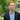# Value vs reference in Javascript

## Types in JavaScript

In JavaScript we have two categories of types.

#### Value types (primitives)

Types that are passed by value:

• String
• Number
• BigInt
• Boolean
• Symbol
• undefined
• null

#### Reference types

Types that are passed by reference:

• Object
• Array
• Function

Let’s have a closer look at both of them.

## Value vs. Reference

When you assign a variable, the JavaScript engine decides whether the value is a primitive or a reference value.

#### Primitive

When we assign a value as primitive, the value is actually stored in the variable. This means that when you manipulate the variable, you are working on the actual value stored in the variable. If you assign primitive variables to other variables using `=`, their values are copied to new variables. We say that they are copied by value.

#### Reference

When we assign non-primitive value to the variable, we copy them by reference. In other words, variables are given a reference to that value, so they don’t actually contain the value.

When you assign a non-primitive variable to other variable using `=`, its reference is copied to the new variable and so they now both point to the same object’s location in memory. Consequently, if you decide to manipulate only one of them, you are actually working on the reference, which means you manipulate both variables!

This is crucial to understand as it’s often the reason behind bugs.

## Examples

#### Primitive values

``````const a = 5
let b = a

console.log(a) // 5
console.log(b) // 5

b = 10

console.log(a) // 5
console.log(b) // 10``````

As you can see in the example above, the value stored in the variable `b` has been changed, but the value of variable `a` remains intact. This is because variables `a` and `b` have no relationship. By copying value from variable `a` to `b`, we created a new independent value.

``````let index = 0

function increaseIndex(index) {
index++
}

increaseIndex(index)
console.log(index) // 0``````

The index value stays 0 even after executing `increaseIndex` function. This is because primitive values are copied by value. We’re dealing with two independent values here, so changing the copied value has no effects on the original value.

#### Non-primitive values

``````const person_one = {
age: 20
}
const person_two = person_one

person_two.name = 'George'

console.log(person_one.name) // 'George'
console.log(person_two.name) // 'George'``````

By changing the name of `person_two`, we’re also modifying value in `person_one` because both `person_one` and `person_two` are pointing to the same object. When the variable `person_two` is created and assigned to `person_one`, we’re creating an alias to the original object, not a new object.

``````const person = {
age: 20
}
function changeName(person) {
person.name = 'George'
}

changeName(person)
console.log(person.name) // 'George'``````

In this case, the `person` name was changed after executing `changeName` function. This is because when we pass an object into the function, we’re passing a reference to that object. When we change a property of that object within the function, the change will be reflected in the outer scope.

### Summary

• Primitives are copied by their value
• Objects are copied by their reference
• When you manipulate variable that stores a primitive value, you are working on the value stored in the variable
• When you manipulate an object, you are working on the reference to that object, not on the actual objectThis website is owned by Mateusz Janusz who is a software developer. He writes here about things that he is interested in or is currently learning.
You can find him also at Github and contact on LinkedIn.